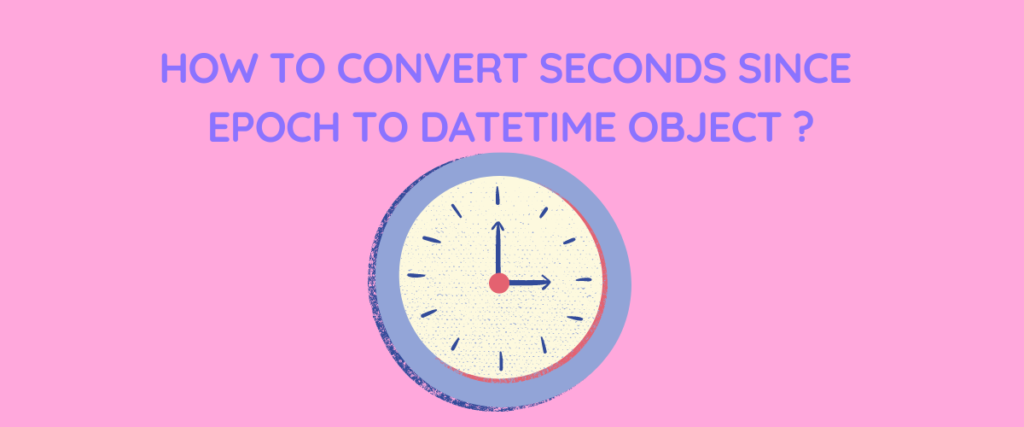# 3 Methods to Convert Seconds – Epoch to a Datetime ObjectEpoch time, also known as Unix or POSIX time, is the standard time most computers use to determine the time after an epoch. Since computers can only understand 0s and 1s, we can’t really expect the computer to understand the date and time format we follow (for example, 01-12-2003).

So the date and time are fed to the computer in terms of seconds, and it is taught to interpret them as normal time (human-readable). A reference point is taken, and the current date is calculated as the number of seconds passed from that reference point.

In this article, we are going to go through epoch time, and how to convert it to a datetime object.

## What Is Epoch Time?

Before we understand what epoch time is, let us first understand what an epoch is.

An epoch with respect to epoch time is the reference point used to determine or calculate how much time has passed. Most programming languages and computer systems use January 1, 1970, 00:00:00 (midnight) as an epoch. This specific timestamp is also called Coordinated Universal Time (UTC).

So to put it simply, epoch time is the number of seconds elapsed or the amount of time passed since the epoch time. For example, if someone says the time is 164531, it simply means that 165431 seconds have passed since epoch time, or January 1, 1970.

Although it may seem like a complicated concept to use a reference point to calculate how much time has passed, there are a few uses for epoch time.

One major reason all developers and programmers prefer epoch time is because of its storage efficiency. Rather than storing the date and time in the form of words like day, month, and year, storing the same date in the form of seconds takes less space, right?

Also, storing the whole date and time in the form of seconds helps in date and time manipulations such as addition and subtraction of dates.

Many operating systems use epoch time to mention the timestamps for the creation and updation of files. Even the time when a file or directory is accessed is represented by epoch time.

Let us see an example of epoch time.

We don’t need to do much. There is a specially built-in module called ‘`time`‘ that gives how many seconds have passed since the epoch.

Let us see the code.

```import time
epochtime = time.time()
print(f"The current epoch time is: {epochtime}")
```

In the first line, we are importing the time module, and in the following line, we are creating a variable called `epochtime` which stores the number of seconds that have passed since the epoch calculated with the help of the `time()` function.

Lastly, we are printing the result.

The output of the code is shown below.

Now that we understand what an epoch time is, let us see how to convert an epoch time to a datetime object.

## Convert Epoch Time to Datetime Object

Again, we do not need to do anything. In the datetime module, we have a few methods that take seconds (epoch time) as input and give out a datetime object. You can find a detailed explanation of the functions used in this example in the official documentation of the datetime module.

### 1. Using datetime.fromtimestamp()

This is the most direct approach to returning a datetime object (YYYY-MM-DD) from POSIX time. Let us see an example.

```from datetime import datetime
sse = 1690974474
dtobject = datetime.fromtimestamp(sse)
print("The datetime object is :",dtobject)
```

We are importing the datetime module in the first line.

Next, we are initializing a variable called `sse`(seconds since epoch) which stores the current epoch time.

The variable `dtobject` stores the results of using the timestamp method on the epoch time, which gives us the format of the date (YYYY-MM_DD) and time(HH:MM:SS).

In the last line, as you can see, we are printing the date time object.

### 2. Using datetime.fromtimestamp() With Time Zone

We use this example as an extension to the first method. In this example, we are also going to include the time zone in the date and time object.

```from datetime import datetime
import pytz
sse = 1690974479
dtobject = datetime.fromtimestamp(sse, pytz.utc)
localtz = pytz.timezone('Asia/Kolkata')
localdt = dtobject.astimezone(localtz)
print(localdt)
```

In this example, we are using `pytz` library that allows accurate and cross-platform timezone calculations using Python 2.4 or higher.

You can use your local time zone in line 5.

The variable `localdt` stores the datetime object computed by the method.

### 3. Using datetime.utcfromtimestamp()

This method is kind of similar to the first method we discussed, with the only difference being how it treats the time zone. While the first method considers the local timezone while returning the datetime object, the `utcfromtimestamp` does not consider the time zone.

The code is given below.

```from datetime import datetime
sse = 1690974476
dtobject = datetime.utcfromtimestamp(sse)
print(dtobject)
```

The code is essentially the same since we have not considered any time zone.

## Summary

To summarize, we have learned what epoch time is and its uses in computers and programming languages, and we have also understood its concept with the help of an example using the `time` module.

Next, we have looked at the possible approaches to converting the epoch time to date time object using the functions of `datetime` module. We looked at three approaches – using `fromtimestamp` without a timezone, also with timezone and `utcfromtimestamp`.

## References

Epoch time Wikipedia

You can find more about the datetime module here

Refer to pytz documentation